Courses

# Capacitance NAT Level - 2

## 10 Questions MCQ Test Topic wise Tests for IIT JAM Physics | Capacitance NAT Level - 2

Description
This mock test of Capacitance NAT Level - 2 for Physics helps you for every Physics entrance exam. This contains 10 Multiple Choice Questions for Physics Capacitance NAT Level - 2 (mcq) to study with solutions a complete question bank. The solved questions answers in this Capacitance NAT Level - 2 quiz give you a good mix of easy questions and tough questions. Physics students definitely take this Capacitance NAT Level - 2 exercise for a better result in the exam. You can find other Capacitance NAT Level - 2 extra questions, long questions & short questions for Physics on EduRev as well by searching above.
*Answer can only contain numeric values
QUESTION: 1

### A leaky parallel plate capacitor is filled completely with a material having dielectric constant K = 5 and electrical conductivity σ = 7.4 × 10–12Ω–1m–1. If the charge on the capacitor at the instant t = 0 is q0 = 8.55µC, then calculate the leakage current (in µA) at the instant t = 12s.

Solution: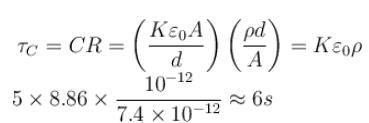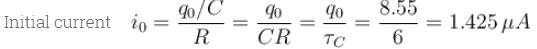Now current as function of time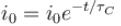or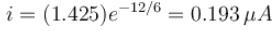*Answer can only contain numeric values
QUESTION: 2

### In the figure shown find the equivalent capacitance between terminals A and B. The letters have their usual meaning capacitance is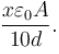Then x is.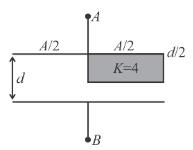Solution: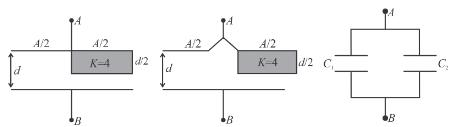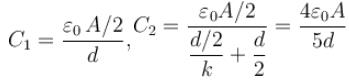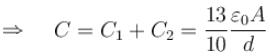*Answer can only contain numeric values
QUESTION: 3

### A capacitor of capacitance 5µF is connected to a source of constant emf of 200 V. Then the switch was shifted to contact 2 from contact 1. Find the amount of heat generated (in mJ) in the  400Ω resistance.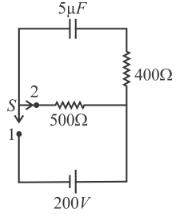Solution:

Potential energy stored in the capacitor,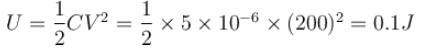During discharging this 0.1J, will distribute in direct ratio of resistance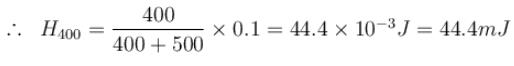*Answer can only contain numeric values
QUESTION: 4

A 8µF capacitor C1 is charged to V0 = 120volt. The charging battery is then removed and the capacitor is connected in parallel to an uncharged +4µF capacitor C2.
What is the stored energy (in units of 10–2 J) before and after the switch S is closed?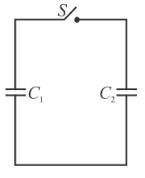Solution: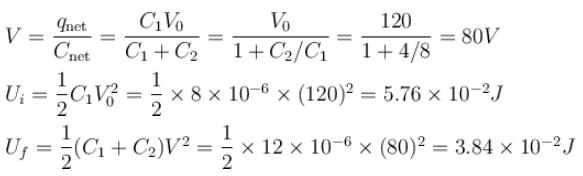*Answer can only contain numeric values
QUESTION: 5

In the circuit shown, a time varying voltage V = 2000t volt is applied where t is second. At time t = 5ms, determine the current (in A) through the resistor R = 4Ω and through the capacitor C = 300µF.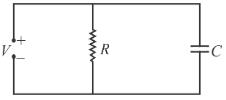Solution:

At t = 5 ms, V = 10 volt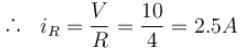Further  q  = CV = (300 × 10–6) (2000t) = 0.6t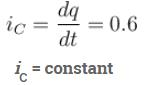*Answer can only contain numeric values
QUESTION: 6

A parallel plate capacitor is to be designed which is to be connected across 1kV potential difference. The dielectric material which is to be filled between the plates has dielectric constant K = 6π and dielectric strength 107V/m. For safely the electric field is never to exceed 10% of the dielectric strength. With such specifications, if we want a capacitor of capacitance 50pF, what minimum area (in mm2) of plates is required for safe working?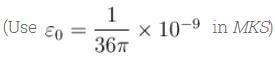Solution: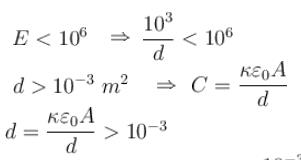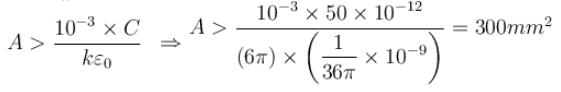*Answer can only contain numeric values
QUESTION: 7

In the figure shown the capacitor is initially uncharged. The current in (R3 = R) at time t is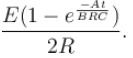Find the value of (A + B)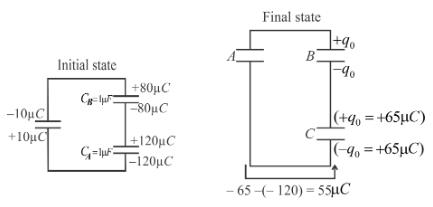Solution:

Applying Kirchoff’s law in
Loop1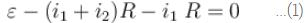Loop 2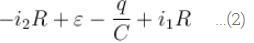eliminating i1 from (1) and (2)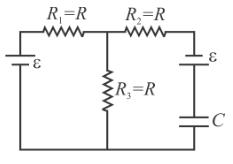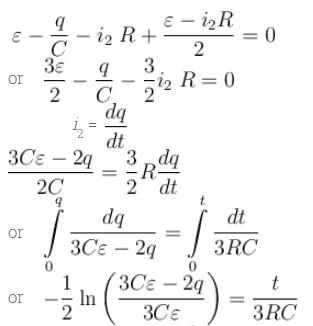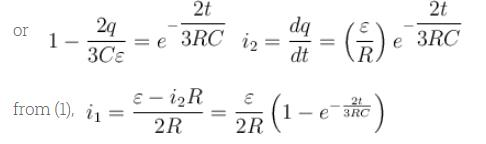A + B =  2 + 3 = 5

*Answer can only contain numeric values
QUESTION: 8

In the circuit shown a charged capacitor C1 = 3µF is discharged through R = 1  by putting the switch is position 1. When the current reaches I0 = 2A, the switch is thrown to position 2 to discharge through uncharged capacitor C2 = 6µF and steady state is allowed to reach. Find the heat dissipated (in Joules) in the resistor R after switch is thrown to position 2.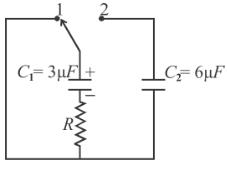Solution:

The charge on the capacitor when current reaches I0
q0 = (I0RC1
When the switch is in position 2, this charge is shared with capacitor C2 and at steady state potential across C1 is equal to that across C2. The energy lost in this process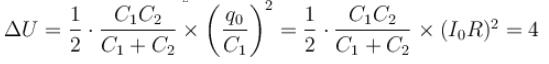*Answer can only contain numeric values
QUESTION: 9

In the circuit shown the capacitors are initially uncharged. In a certain time the capacitor of capacitance 2µF gets a charge 20µC. In that time interval, find the heat produced (in µJ) in each resistor 6Ω.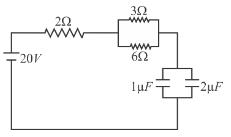Solution: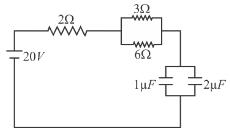Energy taken from cell = 20 × 30 µJ
= 600 µJ
Energy stored in capacitors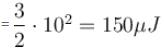∴   Heat produced in resistors = 600 – 150 = 450 µJ
Divide this heat in 2Ω and (equivalent of 3Ω and 6Ω  i.e. 2Ω)
which is 225µJ, 225 µJ
∴  Heat produced in 2Ω = 225µJ
Further divide 225 µJ in 3Ω and 3Ω in inverse ratio of resistance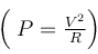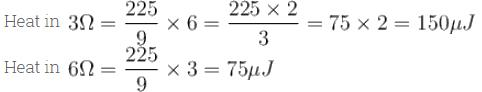Heat (in 2Ω ) 225µJ, Heat (in 2Ω) 150 µJ, Heat (in 2Ω) 75 µJ

*Answer can only contain numeric values
QUESTION: 10

Given that CA = 1µFCB = 2µF, and CC = 2µF, Initially each capacitor was charged to potential differences of VA = 10VVB = 40V and VC = 60V separately and are kept as shown in figure (a). Now they are connected as shown in figure(b). The positive and negative sign shown in figure (b) represent initial polarities. If total amount of heat produced in µJ is given by (3100 – N) by the time steady state is reached find value of N.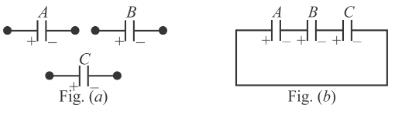Solution: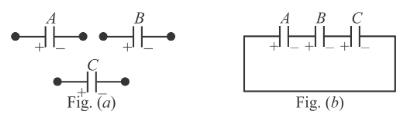q1 – q3 = 10 – 120 = – 110    ...(1)
–q2 + q3 = – 80 + 120 = + 40    ...(2)
In the final state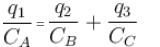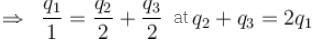Solving we get q3 = 65μC
The charge on lower plate of capacitor CC changes from  –120µC to –65µC
Hence the charge flowing through shown connecting wire is
(120 – 65) = 55 µC
final charges
q3 = 65µC ; q2 = 25µC ; q1 = 45µC
Heat produced = Ui – Uf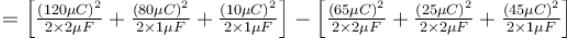= 3025 µJ